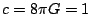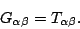Next: Preliminaries Up: The Meaning of Einstein's Equation Previous: The Meaning of Einstein's Equation

# Introduction

General relativity explains gravity as the curvature of spacetime. It's all about geometry. The basic equation of general relativity is called Einstein's equation. In units where, it says(1)

It looks simple, but what does it mean? Unfortunately, the beautiful geometrical meaning of this equation is a bit hard to find in most treatments of relativity. There are many nice popularizations that explain the philosophy behind relativity and the idea of curved spacetime, but most of them don't get around to explaining Einstein's equation and showing how to work out its consequences. There are also more technical introductions which explain Einstein's equation in detail -- but here the geometry is often hidden under piles of tensor calculus.

This is a pity, because in fact there is an easy way to express the whole content of Einstein's equation in plain English. In fact, after a suitable prelude, one can summarize it in a single sentence! One needs a lot of mathematics to derive all the consequences of this sentence, but it is still worth seeing -- and we can work out some of its consequences quite easily.

In what follows, we start by outlining some differences between special and general relativity. Next we give a verbal formulation of Einstein's equation. Then we derive a few of its consequences concerning tidal forces, gravitational waves, gravitational collapse, and the big bang cosmology. In the last section we explain why our verbal formulation is equivalent to the usual one in terms of tensors. This article is mainly aimed at those who teach relativity, but except for the last section, we have tried to make it accessible to students, as a sketch of how the subject might be introduced. We conclude with a bibliography of sources to help teach the subject.

Next: Preliminaries Up: The Meaning of Einstein's Equation Previous: The Meaning of Einstein's Equation

© 2006 John Baez and Emory Bunn